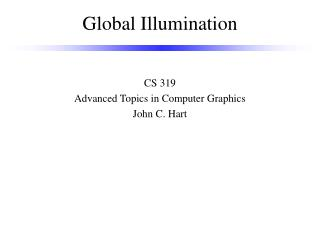DownloadDownload PresentationGlobal Illumination

# Global Illumination

Télécharger la présentation## Global Illumination

- - - - - - - - - - - - - - - - - - - - - - - - - - - E N D - - - - - - - - - - - - - - - - - - - - - - - - - - -
##### Presentation Transcript

1. Global Illumination CS 319 Advanced Topics in Computer Graphics John C. Hart

2. Global Illumination Accounts for all light in a scene Techniques • The Rendering Equation • theoretical basis forlight transport • Path Tracing • attempts to trace“all rays” in a scene • Photon Mapping • deposits light energy on surfaces for later collection • Radiosity • balances diffuse interreflection

3. I(x,x’) – intensity at x from x’ g(x,x’) – geometry term (g) % of light from x’ that reaches x e.g. shadows, occlusion e(x,x’) – emissive term (e) light emitted by x’ toward x e.g. light sources r(x,x’,x’’) – reflectivity % of intensity incident at x’ from x’’ reflected in the x direction x x’ The Rendering Equation x” I(x,x’) I(x’,x”) g(x,x’)

4. Describing Paths I = ge + gR(I) • R() – linear integral “reflection” operator • Reflected intensity is twice the power if the incident intensity is twice the power R(cI) = cR(I) • Reflected intensity from two light sources is equal to the sum of the intensities reflected from each R(I1 + I2) = R(I1) + R(I2) • Solve for intensity I (1 – gR)I = ge I = (1 – gR)-1ge I = ge + gRge + gRgRge + gRgRgRge + ...

5. Reflectance Categories • L – emitter (light source) • E – receiver (eye) • D – diffuse • Ideal r(x,x’,x”) = r(,x’,x”) • In general, any interaction where light is scattered across hemisphere • S – specular • Ideal (e.g. mirror, refraction) r(x,x’,x”) = d(arg(x,x’) – arg(x’,x’’)) • In general, any interaction where light is reflected in a single direction D S

6. Paths • OpenGL LDE LDSE (w/mirror or env. map) I = ge + gDe (no shadows) I = ge + gDge (shadow buffer) • Ray tracing LDS*E I = ge + g(Sg)*Dge • Radiosity LD*E I = g(Dg)*e

7. Energy Transport dw dw • Radiance – power per unit projected area perpendicular to the ray, per unit solid angle in the direction of the ray • Fundamental unit of light transport • Invariant along ray dA dA dA1 dA2 L1 L2 dw1 dw2 d2F1 = L1dw1dA1 = L2dw2dA2 = d2F2 dw1 = dA2/r2, dw2 = dA1/r2 dw1 dA1 = dA1 dA2/r2 = dw2 dA2 L1 = L2

8. Radiance Form of Rendering Equation x’ w q’ w’ • V(x,x’) – visibility term • 1 if visible • 0 if occluded q x

9. Energy Conservation • Energy remains contant Out – In = Emitted – Absorbed • Global conservation • Total energy input must equal total energy output • Where does it go? Mostly heat • Closed environment • Local conservation • Incident energy must be reflected or absorbed • Ratio controlled by Fresnel